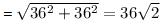Courses

# Test: Motion in a Plane

## 5 Questions MCQ Test Physics Class 11 | Test: Motion in a Plane

Description
This mock test of Test: Motion in a Plane for JEE helps you for every JEE entrance exam. This contains 5 Multiple Choice Questions for JEE Test: Motion in a Plane (mcq) to study with solutions a complete question bank. The solved questions answers in this Test: Motion in a Plane quiz give you a good mix of easy questions and tough questions. JEE students definitely take this Test: Motion in a Plane exercise for a better result in the exam. You can find other Test: Motion in a Plane extra questions, long questions & short questions for JEE on EduRev as well by searching above.
QUESTION: 1

### A stone is thrown upwards with initial velocity of 20 m s-1, the height that stone will reach would be:

Solution:

► Initial velocity = u = 20 m/s
► Final velocity = v = 0     ( at maximum height , v = 0 )
Acceleration due to gravity(g)  in this case , is taken as negative.
This is because when the direction of motion of object is opposite to "g" , then value of g is taken as -ve.
Hence, g = -9.8 m/s²
Let's use the formula:
► v2 - u2 = 2gh
► 02 - 202 = 2 * (- 9.8) * h
► - 400 = -19.6 h
► h = - 400 /(-19.6) = 20.408 m (approximately)

QUESTION: 2

### A particle has an initial velocity of 3i + 4j and an acceleration of 0.4i + 0.3j. Its speed after 10s is:

Solution:

Use equation of kinematics: v = u + at
Initial velocity, u = 3i + 4j
Acceleration, a = 0.4i + 0.3j
Time, t = 10s
► v = (3i + 4j) + 10(0.4i + 0.3j)
► v = (3i + 4j) + (4i + 3j)
► v = 7i + 7j
► |v| = √(7+ 72) = 7√2

QUESTION: 3

### A body sliding on a smooth inclined plane requires 4 seconds to reach the bottom starting from rest at the top. How much time does it take to cover one-fourth distance starting from the rest at the top?

Solution:

A body start from rest so, u = 0
Let total distance covered = s
Let a body moves with accerlation = a
► s = 1/2 × a× t2
► s = 1/2× a × 42
► s = 8a
The one-fourth of total distance, s'  = 8a/4
= 2a
► s' = 1/2 × a × t2
► 2a / a = 1/2 × t2
► 4 = t2
► t = 2 s

QUESTION: 4

A car travelling at 36km/h-1 due North turns West in 5 seconds and maintains the same speed. What is the acceleration of the car?

Solution:

Initial velocity of car = 36 km/hr due north
Final velocity of car = 36 km/hr due west

The magnitude of change in velocity:(since velocity is a vector, so direction has to be taken into account)

Acceleration = (Change in Velocity) / Time

= [36(√2) * 5/18] / 5
= 2(√2) m/s2 in north west

QUESTION: 5

Water drops fall at regular intervals from a tap that is 5m above the ground. The third drop is leaving the tap at the instant the first drop touches the ground. How far above the ground is the second drop at that instant?

Solution:

Time taken by first drop to cover 5 cm (u = 0), is:
► h = 1/2 gt2
► 5 = 1/2 x 10 x t2
► t = 1 sec
Hence interval is 0.5 sec for each drop.
Now distance fallen by second drop in 0.5 sec
► h1 = 1/2 gt2
= 1/2 x 10 x (0.5)2
= 5 x 0.25
= 1.25 m
Height above the ground ( of 2nd drop) = 5 - 1.25 = 3.75 m